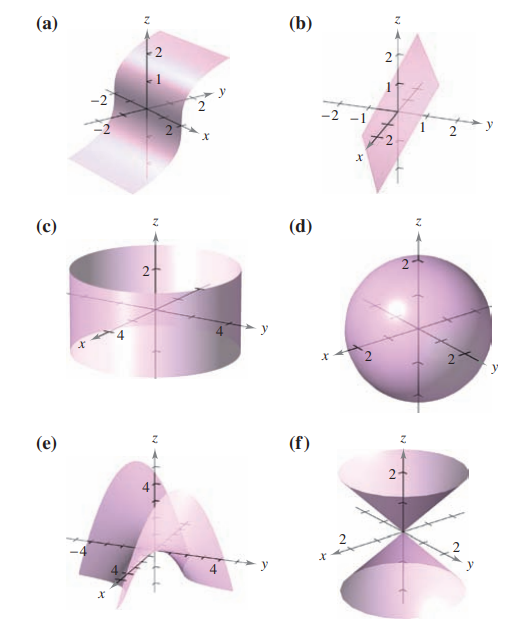×
Get Full Access to Calculus: Early Transcendental Functions - 6 Edition - Chapter 15.5 - Problem 2
Get Full Access to Calculus: Early Transcendental Functions - 6 Edition - Chapter 15.5 - Problem 2

×

# ?In Exercises 1-6, match the vector-valued function with its graph. [The graphs are labeled (a), (b), (c), (d), (e), and (f).]ISBN: 9781285774770 141

## Solution for problem 2 Chapter 15.5

Calculus: Early Transcendental Functions | 6th Edition

• Textbook Solutions
• 2901 Step-by-step solutions solved by professors and subject experts
• Get 24/7 help from StudySoup virtual teaching assistantsCalculus: Early Transcendental Functions | 6th Edition

4 5 1 326 Reviews
11
3
Problem 2

In Exercises 1-6, match the vector-valued function with its graph. [The graphs are labeled (a), (b), (c), (d), (e), and (f).]$$\mathbf{r}(u, v)=u \cos v \mathbf{i}+u \sin v \mathbf{j}+u \mathbf{k}$$

Text Transcription:

r(u, v) = u cos vi + u sin vj + uk

Step-by-Step Solution:

Step 1 of 5) Figure 11.36 Selected points and parameter values on the line in Example 1. The arrows show the direction of increasing t.

Step 2 of 2

## Discover and learn what students are asking

Statistics: Informed Decisions Using Data : Inference about Measures of Central Tendency
?Locate the efficiency of the sign test in Section 15.1 for both large samples and small samples. What do these values indicate?

Unlock Textbook Solution